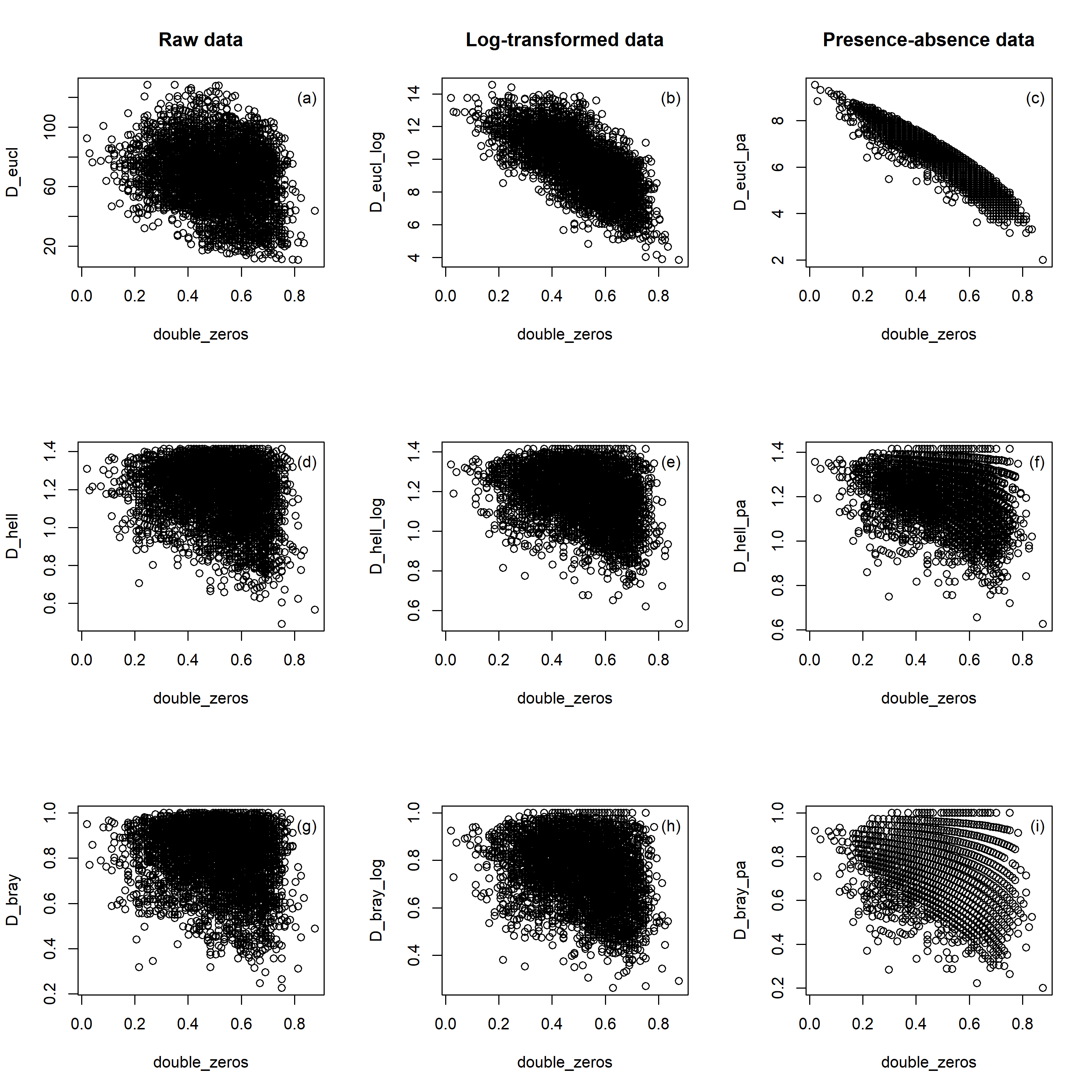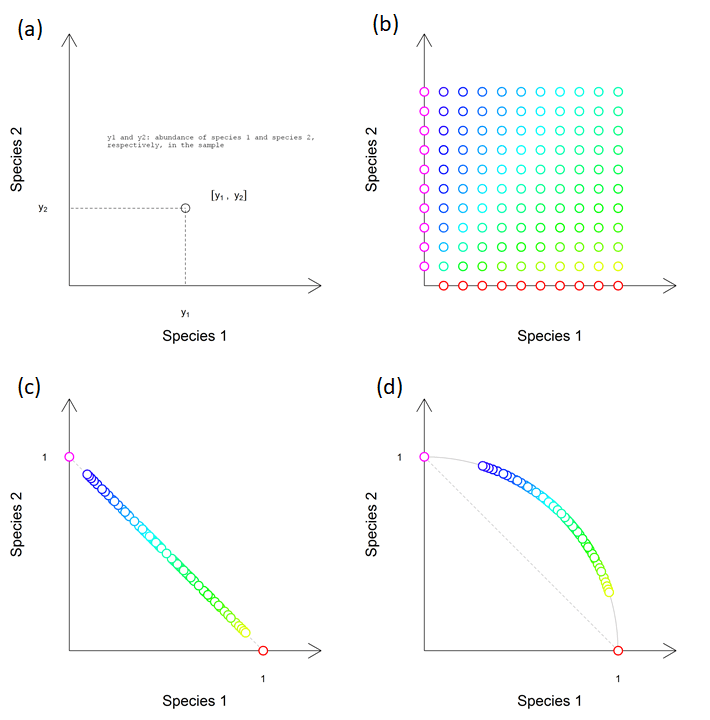# Euclidean distance is sensitive to double zero problem, while Hellinger is not: visualization

One obstacle to analysing community ecology data with linear ordination methods (principal component analysis, redundancy analysis) is their reliance on Euclidean distances to quantify compositional differences among communities (sites). Euclidean distance is known to be sensitive to double zero problem, i.e. species that are missing in the species composition of both compared communities. There exists a reasonable argument that for species composition data (occurrences of species in different communities), the absence of species in both compared communities does not really reveal meaningful information about their ecological resemblance. The species may be missing because the environment of each community is outside of the ecological niche of the species, but then both communities could be at the same end of the gradient (e.g. both too dry for species to occur, and hence environmentally quite similar) or each community on the other end of the gradient (one too dry, the other too wet, hence environmentally quite different). Distances that can be meaningfully applied for species composition data should preferentially ignore double zeros (be “asymmetric” – treat differently double presence and double absence). In the case of relatively homogeneous species composition data (with minimum double zeros) the Euclidean distance can be useful.

In the numerical example below, I will show the comparison of Euclidean and Hellinger distance in the context of the double zero problem. This comparison will show that Euclidean distance is really affected by the double zero problem, and the effect is strongest when the species composition data are transformed (by log or even presence-absence transformation). Hellinger distance, in contrast, is claimed as not sensitive to the double zero problem, and the illustration below clearly illustrates this feature. For comparison, I included also the third distance metric, Bray-Curtis, which is a quantitative version of Sørensen similarity and is known to be asymmetric (ignoring double zeros).

I took an example dataset about forest vegetation sampled in the Vltava river valley (Czech Republic), which is compositionally rather heterogeneous (and therefore contains a large number of double zeros). Dissimilarity (Euclidean, Hellinger and Bray-Curtis) is calculated among each pair of the 97 vegetation plots (communities). Abundances in these plots were either used raw (percentage cover of individual plant species estimated in the field), log-transformed as log (x+1), or transformed into presences and absences. The proportion of double zeros was for each pair of communities calculated as the number of species jointly missing in both compared communities, divided by the number of all species in the whole dataset (i.e. not only species present in the two communities, but all species present in all 97 vegetation plots).

I displayed the relationship of the proportion of double zeros (x-axis) against each dissimilarity metric (y-axis), with Euclidean, Hellinger and Bray-Curtis distance in the upper, middle and lower rows of the figure below, respectively, and applied on data either untransformed (first column), log-transformed (middle column) or transformed into presences-absences (right column). I have not calculated any statistic to quantify the relationship between distances and proportions of double zeros, and haven’t tested it, since individual data points represent pairwise comparisons among pairs of communities in the dataset, and some of them share the same community (and are therefore not independent).

The results show that Euclidean distance is related to the proportion of double zeros in the community, and this relationship is very strong if the original species composition data were transformed into presences-absences (but is also obvious for log-transformed data, less for untransformed). Hellinger distance, in contrast, is not directly related to the proportion of double zeros. In the case of Hellinger distance, the pattern appears a bit triangular: if the proportion of double zeros is low (i.e. the communities share most of the species), calculated dissimilarity intuitively cannot be too low; on the other side, if the double zero proportion is high, the dissimilarity can be both low or high (depending on which species are being shared among communities). Bray-Curtis distance behaves very similarly to the Hellinger distance; in fact, the Hellinger and Bray-Curtis distances are rather correlated, and this correlation is very strong when calculated from presence-absence data (pattern not shown here).The relationship of the dissimilarity between the pair of communities (y-axis, either Euclidean, D_eucl, or Hellinger, D_hell, or Bray-Curtis, D_bray) and the proportion of double zeros in the compared pair of communities (x-axis, double_zero).

In this context, I also focused on Hellinger standardization (or transformation, call it as you wish). The reason is that Hellinger distance between pair of communities can be calculated by taking the raw species composition data (with original or transformed abundances, e.g. by log or sqrt transformation), and applying the Hellinger standardization on it (which involves relativization of abundances within the community by dividing abundance of each species by the sum of abundances in the community, and then by square-rooting the relativized value). If we apply Euclidean distance on such Hellinger standardized raw data, we will get Hellinger distance.

I visualized the process of standardization in the figure below. Panel (a) shows the concept of two-dimensional species space, where each dimension is defined by an abundance of one species (species 1 on the x-axis and species 2 on the y-axis). Communities (circles) are located in this space according to the abundance of the two species. Panel (b) shows the distribution of 120 samples (communities) in the two-species space. Absolute values of the abundances are not relevant, important is that the units on the x- and y-axis are the same; e.g., in the yellow sample which is at the second row from the bottom the most at the right, the species abundances could be [100, 20], [10, 2], or [1, 0.2]. Panel (c) shows the result of the first step in Hellinger standardization, which is the calculation of “species profiles” (species abundances is standardized by row sums, i.e. the sum of species abundances in its community). Samples standardized to species profiles are located on the hypotenuse of the right-angled triangle with corner coordinates [0,0], [0,1] and [1,0]. Panel (d) shows the position of samples after the second step of Hellinger standardization, square rooting of species profiles; all samples are located at the perimeter of the circle, which has a radius of 1.

What is interesting on Hellinger-standardized abundance values is that communities with the same colour in the original two-species space have the same abundance after standardization.Visualization of Hellinger standardization. (a) Position of the community sample in the two-dimensional space defined by species 1 and species 2 is defined by the abundances of these species in the community. (b) Distribution of 120 communities with different abundances of species 1 and species 2. (c) Position of communities after standardization to “species profiles” (first step of Hellinger standardization) and (d) square-rooting (the second step of the standardization). The animation of the whole process can be seen here: https://vimeo.com/689244325/339fd4c86e

## 1 thought on “Euclidean distance is sensitive to double zero problem, while Hellinger is not: visualization”

1.David S. Mason

I love the materials you’ve put online. You are doing a service!